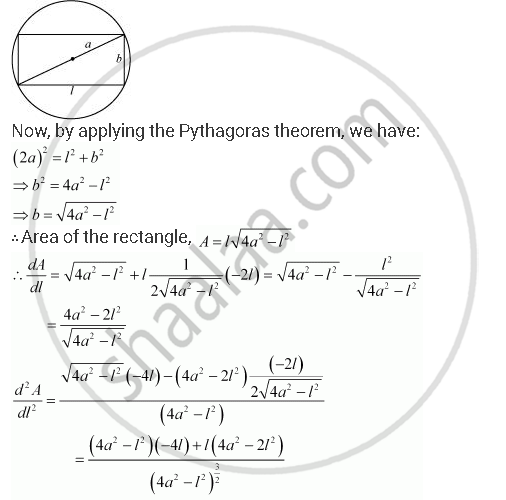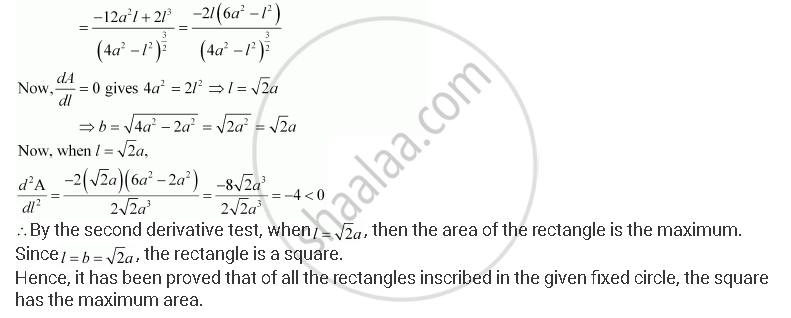Share

# Show that of All the Rectangles Inscribed in a Given Fixed Circle, the Square Has the Maximum Area. - CBSE (Commerce) Class 12 - Mathematics

#### Question

Show that of all the rectangles inscribed in a given fixed circle, the square has the maximum area.

#### Solution

Let a rectangle of length l and breadth b be inscribed in the given circle of radius a.

Then, the diagonal passes through the centre and is of length 2a cm.Is there an error in this question or solution?

#### APPEARS IN

NCERT Solution for Mathematics Textbook for Class 12 (2018 to Current)
Chapter 6: Application of Derivatives
Q: 19 | Page no. 233

#### Video TutorialsVIEW ALL 

Solution Show that of All the Rectangles Inscribed in a Given Fixed Circle, the Square Has the Maximum Area. Concept: Maxima and Minima.
S